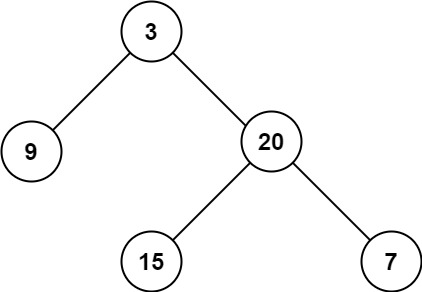# LeetCode: 104. Maximum Depth of Binary Tree

## 题目¶

Given the root of a binary tree, return its maximum depth.

A binary tree's maximum depth is the number of nodes along the longest path from the root node down to the farthest leaf node.

Example 1:```Input: root = [3,9,20,null,null,15,7]
Output: 3
```

Example 2:

```Input: root = [1,null,2]
Output: 2
```

Example 3:

```Input: root = []
Output: 0
```

Example 4:

```Input: root = 
Output: 1
```

Constraints:

• The number of nodes in the tree is in the range [0, 104].
• -100 <= Node.val <= 100

## 解法¶

```# Definition for a binary tree node.
# class TreeNode:
#     def __init__(self, val=0, left=None, right=None):
#         self.val = val
#         self.left = left
#         self.right = right
class Solution:
def maxDepth(self, root):
if root is None:
return 0
return 1 + max(self.maxDepth(root.left), self.maxDepth(root.right))
```